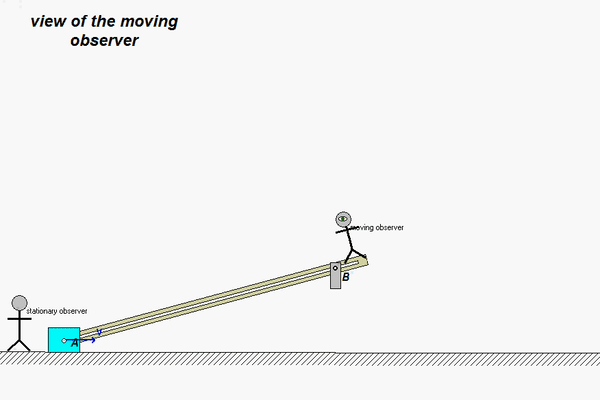# Homework H3.D - Sp23

 Problem statement Solution videoDiscussion and hints:

Let's first take a look at the motion of the mechanism, as shown in the simulation results below for the view of a stationary observer standing on ground.Point B is fixed as the slot in the bar moves over this fixed pin; that is,

vB = a= 0

Now, let's attach an observer to the link. Keep in mind that this observer is unaware that they are moving. This observer actually sees motion for pin B as shown in the animation below showing the view of this observer.This relative motion along the slot is in the x-direction:

(vB/A)rel = vrel = d_dot i

(aB/A)rel = arel i = d_ddot i

where d_dot and d_ddot are unknowns (you will solve for these in your analysis).

Use the above results in the moving reference frame velocity and acceleration equations in order to determine the angular velocity and angular acceleration of the link, as well as d_dot and d_ddot.

Any questions??